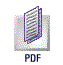Vol.2, No 10, 2000 pp. 1453-1454
UDC 62-133.4+62-253(045)
DYNAMICS OF MACHINES WITH VARIABLE MASS
by L. Cvetičanin
Publisher: Gordon and Breach Science Publishers, 1998

This book is designed to be a complete and integrated text on the dynamic properties of machines, mechanisms and rotors with variable mass. New results are presented from investigations based on the general dynamics of systems with variable parameters. The book considers both weak and strong nonlinear vibrations of these systems and chaotic phenomena are also discussed. The conservation laws and adiabatic invariants for systems with variable mass are formulated and the stability and instability conditions of motion are defined.
The book contains seven chapters for which brief description are given below.
Chapter 1 describes various machines and rotors with time variable mass and parameters such as centrifuges, sieves for sorting particles, transportation mechanisms, lifting mechanisms, cranes, automatic weight measuring instruments, and rotors used in the textile, cable and paper industries.
Chapter 2 introduces modelling of machines with variable mass. The classical laws of mechanics for constant mass are transformed for systems with variable mass. The theory of rigid body is specially treated. The Lagrange procedure for forming differential equations of motion for systems with variable mass is developed.
Chapter 3 and 4 consider the oscillations of machines with variable mass. Nonlinear oscillations of systems with time variable parameters are denoted analytically and compared with numerical results. Systems considered are not only those with small nonlinearity but also those with strong nonlinearity. Differential equations with a complex deflection function are examined, as they describe the motion of the majority of rotors with variable mass. The methods adopted for solving such equations are the Bogolubov-Mitropolski method, the elliptic-Krylov-Bogolubov method, the method of slow variable amplitude and phase, and the method of multiple scales. The influence of reactive force on the motion of the machine is investigated. It can be seen that reactive force has a fundamental influence on dynamic behavior of rotors and mechanisms with variable mass. Therefore, it cannot be neglected.
Chapter 5 treats the problem of chaos in nonlinear systems with variable mass. The analytical Melnikov criteria are extended for such systems. Two examples of real machines are considered: chaos in the crane mechanism and chaos in the separator.
Chapter 6 is about the formulation of conservation laws and adiabatic invariants for systems with variable mass. The first integrals are obtained using the theory of Emmy Noether. Examples of a rotor with variable mass and of a sieve for separating particles are also discussed. The adiabatic invariants for systems with one- and two-degrees-of-freedom for different types of no linearity are also considered. The adiabatic invariants are applied for finding approximate analytical solutions for nonlinear differential equations of motion.
Chapter 7 deals with problems of stability in systems with variable mass. The direct Lyapunov theorems of stability, asymptotic stability and instability are applied. Some examples of Lyapunov function formation for systems with variable mass are shown. In addition some new theories of stability based on the Lyapunov theory are presented and in spite of some limitations, they are simpler than previous procedures and thus much more convenient for engineers. The influence of reactive force on the stability properties of the system is also analyzed.
This book aims to expand previous knowledge and is intended for engineers and investigators dealing in the fields of mathematics, mechanics and physics with the problems of real machines with variable mass.

Prof. Dr. Djordje Djukić, corresponding member
of Serbian Academy of Sciences and Arts